# 如何在 MetaTrader 5 中利用 DirectX 创建 3D 图形

29 四月 2020, 13:07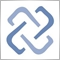0
1 559

### 物体建模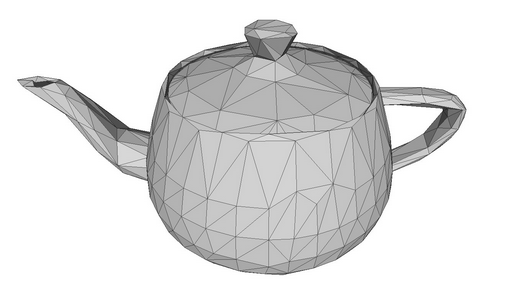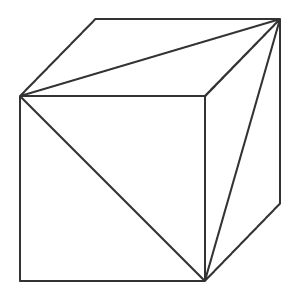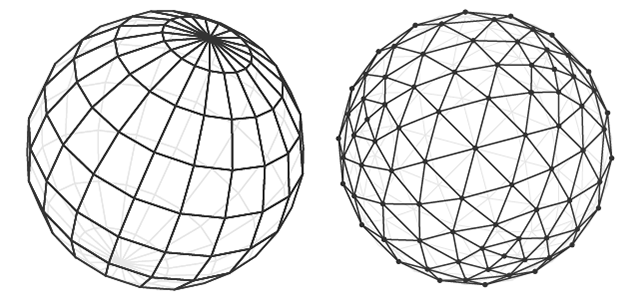### 造型

```//+------------------------------------------------------------------+
//| 应用窗体                                                           |
//+------------------------------------------------------------------+
class CCanvas3DWindow
{
protected:
CCanvas3D         m_canvas;
//--- 画布尺寸
int               m_width;
int               m_height;
//--- 立方体
CDXBox            m_box;

public:
CCanvas3DWindow(void) {}
~CCanvas3DWindow(void) {m_box.Shutdown();}
//-- 创建场景
virtual bool      Create(const int width,const int height){}
//--- 计算场景
void              Redraw(){}
//--- 响应图表事件
void              OnChartChange(void) {}
};

```

1. 30 度视角（M_PI/6），从这处我们观看 3D 场景
2. 纵横比（宽度与高度比率）
3. 距剪切面近处（0.1f）到远处（100.f）的距离

```   //+------------------------------------------------------------------+
//| 创建                                                             |
//+------------------------------------------------------------------+
virtual bool      Create(const int width,const int height)
{
//--- 保存画布维度
m_width=width;
m_height=height;
//--- 为渲染 3D 场景而创建画布
ResetLastError();
if(!m_canvas.CreateBitmapLabel("3D Sample_1",0,0,m_width,m_height,COLOR_FORMAT_ARGB_NORMALIZE))
{
Print("Error creating canvas: ",GetLastError());
return(false);
}
//--- 设置投影矩阵参数-视角、纵横比、与剪裁面的近端和远端距离
m_canvas.ProjectionMatrixSet((float)M_PI/6,(float)m_width/m_height,0.1f,100.0f);
//--- 创建立方体 - 将立方体的两个对角坐标、资源管理器、场景参数传递给它
if(!m_box.Create(m_canvas.DXDispatcher(),m_canvas.InputScene(),DXVector3(-1.0,-1.0,5.0),DXVector3(1.0,1.0,7.0)))
{
m_canvas.Destroy();
return(false);
}
//--- 在场景里加入立方体
//--- 刷新场景
Redraw();
//--- 成功
return(true);
}

```

```   for(int i=20; i<24; i++)
vertices[i].normal=DXVector4(0.0,-1.0,0.0,0.0);

```

```//--- 纹理坐标
for(int i=0; i<faces; i++)
{
vertices[i*4+0].tcoord=DXVector2(0.0f,0.0f);
vertices[i*4+1].tcoord=DXVector2(1.0f,0.0f);
vertices[i*4+2].tcoord=DXVector2(1.0f,1.0f);
vertices[i*4+3].tcoord=DXVector2(0.0f,1.0f);
}

```

4 个切面顶点中的每一个都设置了 4 个角度的纹理映射。 这意味着每个立方体切面在渲染纹理时都会映射一小队结构。 当然，只在设置纹理的情况下才需要这样做。

### 场景计算与渲染

• 在全系坐标中计算每个物体的中心
• 计算物体每个元素的位置，即每个顶点的位置
• 确定像素深度，及其视野的可见性
• 按指定顶点计算每个像素在多边形上的位置
• 根据指定的纹理设置多边形上每个像素的颜色
• 计算光照像素的方向，及其反射
• 为每个像素施加散射光
• 将全系坐标转换为相机坐标
• 将相机坐标转换为投影矩阵上的坐标

```   //+------------------------------------------------------------------+
//| 刷新场景                                                           |
//+------------------------------------------------------------------+
void              Redraw()
{
//--- 计算 3D 场景
m_canvas.Render(DX_CLEAR_COLOR|DX_CLEAR_DEPTH,ColorToARGB(clrBlack));
//--- 根据当前场景更新画布上的图片
m_canvas.Update();
}

```

```   //+------------------------------------------------------------------+
//| 处理图表变化事件                                                    |
//+------------------------------------------------------------------+
void              OnChartChange(void)
{
//--- 获取当前图标尺寸
int w=(int)ChartGetInteger(0,CHART_WIDTH_IN_PIXELS);
int h=(int)ChartGetInteger(0,CHART_HEIGHT_IN_PIXELS);
//--- 根据图表尺寸更新画布尺寸
if(w!=m_width || h!=m_height)
{
m_width =w;
m_height=h;
//--- 调整画布尺寸
m_canvas.Resize(w,h);
DXContextSetSize(m_canvas.DXContext(),w,h);
//--- 根据画布尺寸更新投影矩阵
m_canvas.ProjectionMatrixSet((float)M_PI/6,(float)m_width/m_height,0.1f,100.0f);
//--- 重新计算 3D 场景，并在画布上渲染
Redraw();
}
}

```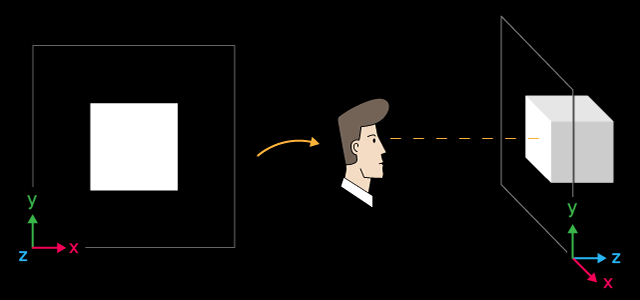X 轴指向右侧，Y 轴指向上方，Z 轴指向 3D 场景内里。 这种坐标系称为左手系。

### 围绕 Z 轴和视角的物体旋转

```   //+------------------------------------------------------------------+
//| 计时器响应                                                         |
//+------------------------------------------------------------------+
void              OnTimer(void)
{
//--- 计算旋转角度的变量
static ulong last_time=0;
static float angle=0;
//--- 获取当前时间
ulong current_time=GetMicrosecondCount();
//--- 计算增量
float deltatime=(current_time-last_time)/1000000.0f;
if(deltatime>0.1f)
deltatime=0.1f;
//--- 立方体绕 Z 轴的旋转角度递增
angle+=deltatime;
//--- 记住时间
last_time=current_time;
//--- 设置立方体绕 Z 轴的旋转角度
DXMatrix rotation;
DXMatrixRotationZ(rotation,angle);
m_box.TransformMatrixSet(rotation);
//--- 重新计算 3D 场景，并在画布上渲染
Redraw();
}

```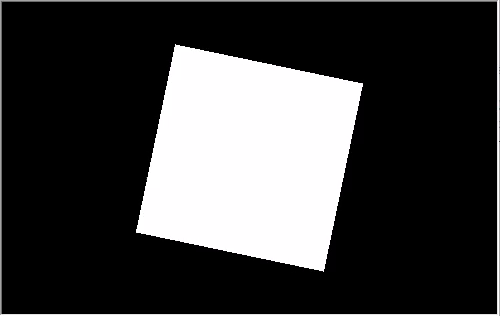```      //--- 设置投影矩阵参数-视角、纵横比、与剪裁面的近端和远端距离
m_matrix_view_angle=(float)M_PI/5;
m_canvas.ProjectionMatrixSet(m_matrix_view_angle,(float)m_width/m_height,0.1f,100.0f);
//--- 创建立方体 - 将立方体的两个对角坐标、资源管理器、场景参数传递给它

```

### 相机位置管理

CCanvas3D 类拥有三个设置重要 3D 场景参数的方法，这些方法相互关联：

```   //+------------------------------------------------------------------+
//| 创建                                                              |
//+------------------------------------------------------------------+
virtual bool      Create(const int width,const int height)
{
....
//--- 在场景里加入立方体
//--- 设置场景参数
m_canvas.ViewUpDirectionSet(DXVector3(0,1,0));  // set the direction vector up, along the Y axis
m_canvas.ViewPositionSet(DXVector3(0,0,0));     // set the viewpoint from the center of coordinates
m_canvas.ViewTargetSet(DXVector3(0,0,6));       // set the gaze point at center of the cube
//--- 刷新场景
Redraw();
//--- 成功
return(true);
}

```

```   //+------------------------------------------------------------------+
//| 计时器响应                                                         |
//+------------------------------------------------------------------+
void              OnTimer(void)
{
//--- 计算旋转角度的变量
static ulong last_time=0;
static float max_angle=(float)M_PI/30;
static float time=0;
//--- 获取当前时间
ulong current_time=GetMicrosecondCount();
//--- 计算增量
float deltatime=(current_time-last_time)/1000000.0f;
if(deltatime>0.1f)
deltatime=0.1f;
//--- 立方体绕 Z 轴的旋转角度递增
time+=deltatime;
//--- 记住时间
last_time=current_time;
//--- 设置绕 Z 轴的旋转角度
DXVector3 direction=DXVector3(0,1,0);     // initial direction of the top
DXMatrix rotation;                        // rotation vector
//--- 计算旋转矩阵
DXMatrixRotationZ(rotation,float(MathSin(time)*max_angle));
DXVec3TransformCoord(direction,direction,rotation);
m_canvas.ViewUpDirectionSet(direction);   // set the new direction of the top
//--- 重新计算 3D 场景，并在画布上渲染
Redraw();
}

```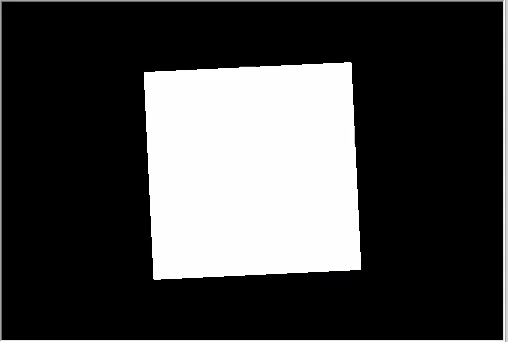### 物体颜色管理

```   //+------------------------------------------------------------------+
//| 创建                                                              |
//+------------------------------------------------------------------+
virtual bool      Create(const int width,const int height)
{
...
//--- 创建立方体 - 将立方体的两个对角坐标、资源管理器、场景参数传递给它
if(!m_box.Create(m_canvas.DXDispatcher(),m_canvas.InputScene(),DXVector3(-1.0,-1.0,-1.0),DXVector3(1.0,1.0,1.0)))
{
m_canvas.Destroy();
return(false);
}
//--- 设置颜色
m_box.DiffuseColorSet(DXColor(0.0,0.5,1.0,1.0));
//--- 在场景里加入立方体
//--- 设置相机的位置，凝视点和顶部的方向
m_canvas.ViewUpDirectionSet(DXVector3(0.0,1.0,0.0));  // 沿 Y 轴方向设置向上顶点
m_canvas.ViewPositionSet(DXVector3(3.0,2.0,-5.0));    // 将相机设置在立方体的右侧、顶部和前面
m_canvas.ViewTargetSet(DXVector3(0,0,0));             // 将凝视点方向设置在立方体的中心
//--- 刷新场景
Redraw();
//--- 成功
return(true);
}

```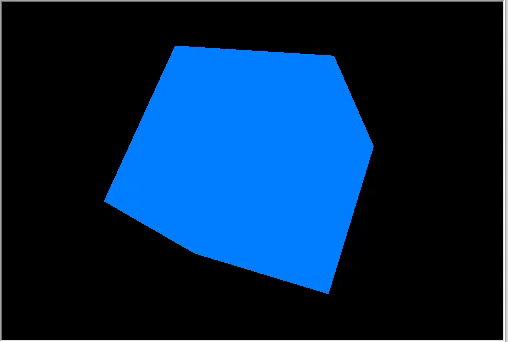### 旋转与运动

```   //+------------------------------------------------------------------+
//| 创建                                                              |
//+------------------------------------------------------------------+
virtual bool      Create(const int width,const int height)
{
...
m_canvas.ProjectionMatrixSet(m_matrix_view_angle,(float)m_width/m_height,0.1f,100.0f);
//--- 将相机放置在坐标中心的顶部和前面
m_canvas.ViewPositionSet(DXVector3(0.0,2.0,-5.0));
m_canvas.ViewTargetSet(DXVector3(0.0,0.0,0.0));
m_canvas.ViewUpDirectionSet(DXVector3(0.0,1.0,0.0));
//--- 创建立方体 - 将立方体的两个对角坐标、资源管理器、场景参数传递给它
if(!m_box.Create(m_canvas.DXDispatcher(),m_canvas.InputScene(),DXVector3(-1.0,-1.0,-1.0),DXVector3(1.0,1.0,1.0)))
{
m_canvas.Destroy();
return(false);
}
//--- 设置立方体颜色
m_box.DiffuseColorSet(DXColor(0.0,0.5,1.0,1.0));
//--- 计算立方体位置和变换矩阵
DXMatrix rotation,translation;
//--- 沿 X、Y和 Z 轴顺序旋转立方体
DXMatrixRotationYawPitchRoll(rotation,(float)M_PI/4,(float)M_PI/3,(float)M_PI/6);
//-- 向右/向下/向内移动立方体
DXMatrixTranslation(translation,1.0,-2.0,5.0);
//--- 取旋转和变化的乘积作为变换矩阵
DXMatrix transform;
DXMatrixMultiply(transform,rotation,translation);
//--- 设置变换矩阵
m_box.TransformMatrixSet(transform);
//--- 在场景里加入立方体
//--- 刷新场景
Redraw();
//--- 成功
return(true);
}

```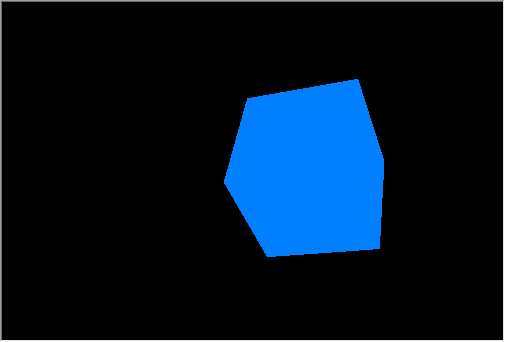### 光照处理

• DirectionLight — 在 CCanvas3D 中设置定向光照的方向
• AmbientLight — 在 CCanvas3D 中设置环境光照的颜色和强度
• DiffuseColor — 在 CDXMesh 及其子类中设置计算出的漫射光照成份
• EmissionColor — 在 CDXMesh 及其子类中设置背景光照成份
• SpecularColor — 在 CDXMesh 及其子类中设置镜面反射成份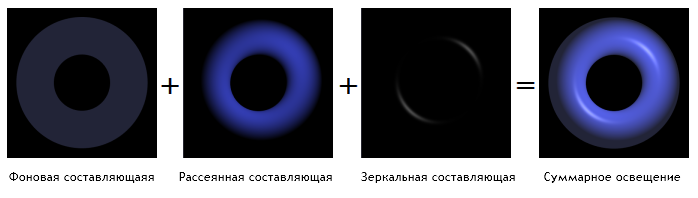Phong 着色模型

1. 将立方体返回到坐标中心。
2. 为其设置白色。
3. 添加黄色定向光源，从上到下照亮场景。
4. 非定向光照设置为蓝色。
```      //--- 将光源设置为黄色，并从上方照向下方定向
m_canvas.LightColorSet(DXColor(1.0,1.0,0.0,0.8f));
m_canvas.LightDirectionSet(DXVector3(0.0,-1.0,0.0));
//--- 设置环境光为蓝色
m_canvas.AmbientColorSet(DXColor(0.0,0.0,1.0,0.4f));
//--- 创建立方体 - 将立方体的两个对角坐标、资源管理器、场景参数传递给它
if(!m_box.Create(m_canvas.DXDispatcher(),m_canvas.InputScene(),DXVector3(-1.0,-1.0,-1.0),DXVector3(1.0,1.0,1.0)))
{
m_canvas.Destroy();
return(false);
}
//--- 设置立方体为白色
m_box.DiffuseColorSet(DXColor(1.0,1.0,1.0,1.0));
//--- 为立方体添加绿色光辉（散射光）
m_box.EmissionColorSet(DXColor(0.0,1.0,0.0,0.2f));

```

`m_canvas.LightDirectionSet(DXVector3(0.0,-1.0,0.0));`

```      //--- 将光源设置为黄色，并从上方照向下方定向
m_canvas.LightColorSet(DXColor(1.0,1.0,0.0,0.8f));
m_canvas.LightDirectionSet(DXVector3(0.0,-1.0,0.0));
//--- 设置环境光为蓝色
m_canvas.AmbientColorSet(DXColor(0.0,0.0,1.0,0.4f));  // must be specified

```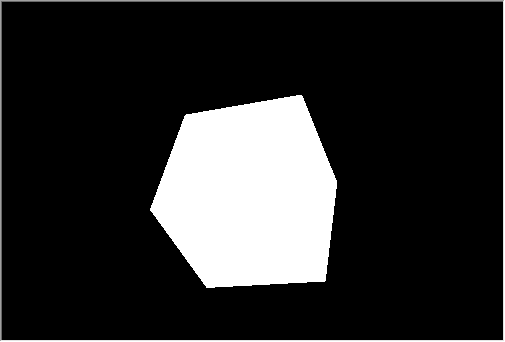### 动画

```int OnInit()
{
...
//--- 创建画布
ExtAppWindow=new CCanvas3DWindow();
if(!ExtAppWindow.Create(width,height))
return(INIT_FAILED);
//--- 设置计时器
EventSetMillisecondTimer(10);
//---
return(INIT_SUCCEEDED);
}

```

```   //+------------------------------------------------------------------+
//| 计时器响应                                                         |
//+------------------------------------------------------------------+
void              OnTimer(void)
{
static ulong last_time=0;
static float time=0;
//--- 获取当前时间
ulong current_time=GetMicrosecondCount();
//--- 计算增量
float deltatime=(current_time-last_time)/1000000.0f;
if(deltatime>0.1f)
deltatime=0.1f;
//--- 流逝时间值递增
time+=deltatime;
//--- 记住时间
last_time=current_time;
//--- 计算立方体位置和旋转矩阵
DXMatrix rotation,translation,scale;
DXMatrixRotationYawPitchRoll(rotation,time/11.0f,time/7.0f,time/5.0f);
DXMatrixTranslation(translation,(float)sin(time/3),0.0,0.0);
//--- 沿坐标轴计算立方体的压缩/扩展
DXMatrixScaling(scale,1.0f+0.5f*(float)sin(time/1.3f),1.0f+0.5f*(float)sin(time/1.7f),1.0f+0.5f*(float)sin(time/1.9f));
//--- 将矩阵相乘从而获得最终变换
DXMatrix transform;
DXMatrixMultiply(transform,scale,rotation);
DXMatrixMultiply(transform,transform,translation);
//--- 设置变换矩阵
m_box.TransformMatrixSet(transform);
//--- 计算光源绕 Z 轴的旋转
DXMatrixRotationZ(rotation,deltatime);
DXVector3 light_direction;
//--- 获取光源的当前方向
m_canvas.LightDirectionGet(light_direction);
//--- 计算并设置光源的新方向
DXVec3TransformCoord(light_direction,light_direction,rotation);
m_canvas.LightDirectionSet(light_direction);
//--- 重新计算 3D 场景，并将其绘制在画布中
Redraw();
}

```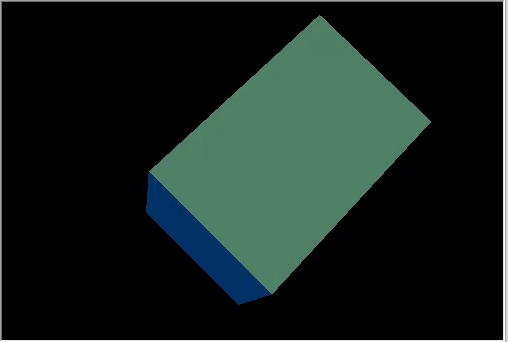### 用鼠标控制相机位置

```int OnInit()
{
...
//--- 设置计时器
EventSetMillisecondTimer(10);
//--- 启用接收鼠标事件：移动和单击按钮
ChartSetInteger(0,CHART_EVENT_MOUSE_MOVE,1);
ChartSetInteger(0,CHART_EVENT_MOUSE_WHEEL,1)
//---
return(INIT_SUCCEEDED);
}
void OnDeinit(const int reason)
{
//--- 删除计时器
EventKillTimer();
//--- 禁止接收鼠标事件
ChartSetInteger(0,CHART_EVENT_MOUSE_MOVE,0);
ChartSetInteger(0,CHART_EVENT_MOUSE_WHEEL,0);
//--- 删除物体
delete ExtAppWindow;
//--- 图表返回到通常的价格显示模式
ChartSetInteger(0,CHART_SHOW,true);
}
void OnChartEvent(const int id,const long &lparam,const double &dparam,const string &sparam)
{
...
//--- 图表变更事件
if(id==CHARTEVENT_CHART_CHANGE)
ExtAppWindow.OnChartChange();
//--- 鼠标移动事件
if(id==CHARTEVENT_MOUSE_MOVE)
ExtAppWindow.OnMouseMove((int)lparam,(int)dparam,(uint)sparam);
//--- 鼠标滚轮滚动事件
if(id==CHARTEVENT_MOUSE_WHEEL)
ExtAppWindow.OnMouseWheel(dparam);

```

```   //+------------------------------------------------------------------+
//| 鼠标移动响应                                                       |
//+------------------------------------------------------------------+
void              OnMouseMove(int x,int y,uint flags)
{
//--- 鼠标左键
if((flags&1)==1)
{
//--- 没有鼠标之前位置的信息
if(m_mouse_x!=-1)
{
//--- 更改位置后更新相机角度
m_camera_angles.y+=(x-m_mouse_x)/300.0f;
m_camera_angles.x+=(y-m_mouse_y)/300.0f;
//--- 将垂直角度设置在（-Pi/2,Pi2）之间的范围内
if(m_camera_angles.x<-DX_PI*0.49f)
m_camera_angles.x=-DX_PI*0.49f;
if(m_camera_angles.x>DX_PI*0.49f)
m_camera_angles.x=DX_PI*0.49f;
//--- 更新相机位置
UpdateCameraPosition();
}
//--- 保存鼠标位置
m_mouse_x=x;
m_mouse_y=y;
}
else
{
//--- 如果未按下鼠标左键，则重置保存的位置
m_mouse_x=-1;
m_mouse_y=-1;
}
}

```

```   //+------------------------------------------------------------------+
//| 鼠标滚轮事件响应                                                    |
//+------------------------------------------------------------------+
void              OnMouseWheel(double delta)
{
//--- 随鼠标滚动更新相机与中心的距离
m_camera_distance*=1.0-delta*0.001;
//--- 将距离设置在 [3,50] 之间
if(m_camera_distance>50.0)
m_camera_distance=50.0;
if(m_camera_distance<3.0)
m_camera_distance=3.0;
//--- 更新相机位置
UpdateCameraPosition();
}

```

```   //+------------------------------------------------------------------+
//| 刷新相机位置                                                       |
//+------------------------------------------------------------------+
void              UpdateCameraPosition(void)
{
//--- 照相机的位置，参考了到坐标中心的距离
DXVector4 camera=DXVector4(0.0f,0.0f,-(float)m_camera_distance,1.0f);
//--- 相机绕 X 轴旋转
DXMatrix rotation;
DXMatrixRotationX(rotation,m_camera_angles.x);
DXVec4Transform(camera,camera,rotation);
//--- 相机绕 Y 轴旋转
DXMatrixRotationY(rotation,m_camera_angles.y);
DXVec4Transform(camera,camera,rotation);
//--- 将相机摆放到位置
m_canvas.ViewPositionSet(DXVector3(camera));
}

```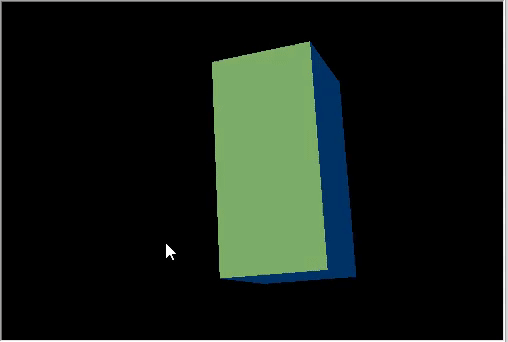### 应用纹理

CDXMesh 及其子类允许指定纹理。 在标准像素着色器中，纹理会与 DiffuseColor 一起使用。 删除对象动画，并应用石头纹理。 它应该位于终端工作目录的 MQL5\Files 文件夹当中：

```   virtual bool      Create(const int width,const int height)
{
...
//--- 非定向光照设为白色
m_box.DiffuseColorSet(DXColor(1.0,1.0,1.0,1.0));

//--- 在方体表面绘制纹理
m_box.TextureSet(m_canvas.DXDispatcher(),"stone.bmp");
//--- 在场景里加入立方体
//--- 刷新场景
Redraw();
//--- 成功
return(true);
}

```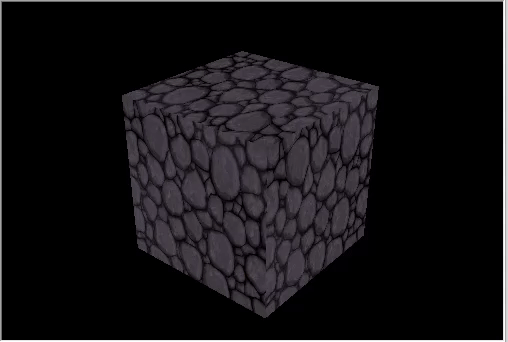### 创建自定义物体

```struct DXVertex
{
DXVector4         position;  // 顶点坐标
DXVector4         normal;    // 法线顶点
DXVector2         tcoord;    // 施加纹理的表面坐标
DXColor           vcolor;    // 颜色
};

```

MQL5\Include\Canvas\DXDXUtils.mqh 辅助类型包含一套生成基本几何（顶点和索引）图元的方法，以及从 .OBJ 文件里加载 3D 几何图元的方法。

```   virtual bool      Create(const int width,const int height)
{
...
// --- 手动创建物体的顶点和索引
DXVertex vertices[];
uint indices[];
//--- 准备球面的顶点和索引
if(!DXComputeSphere(0.3f,50,vertices,indices))
return(false);
//--- 顶点设置为白色
DXColor white=DXColor(1.0f,1.0f,1.0f,1.0f);
for(int i=0; i<ArraySize(vertices); i++)
vertices[i].vcolor=white;
//--- 创建球面物体
if(!m_sphere.Create(m_canvas.DXDispatcher(),m_canvas.InputScene(),vertices,indices))
{
m_canvas.Destroy();
return(false);
}
//--- 设置球面的漫反射颜色
m_sphere.DiffuseColorSet(DXColor(0.0,1.0,0.0,1.0));
//--- 设置镜面反射颜色为白色
m_sphere.SpecularColorSet(white);
m_sphere.TextureSet(m_canvas.DXDispatcher(),"stone.bmp");
//--- 将球面添加到场景
//--- 为环面准备顶点和索引
if(!DXComputeTorus(0.3f,0.1f,50,vertices,indices))
return(false);
//--- 顶点设置为白色
for(int i=0; i<ArraySize(vertices); i++)
vertices[i].vcolor=white;
//--- 创建环面物体
if(!m_torus.Create(m_canvas.DXDispatcher(),m_canvas.InputScene(),vertices,indices))
{
m_canvas.Destroy();
return(false);
}
//--- 为环面设置漫反射颜色
m_torus.DiffuseColorSet(DXColor(0.0,0.0,1.0,1.0));
m_torus.SpecularColorSet(white);
m_torus.TextureSet(m_canvas.DXDispatcher(),"stone.bmp");
//--- 将环面添加到场景
//--- 刷新场景
Redraw();
//--- 成功
return(true);
}

```

```   void              OnTimer(void)
{
...
m_canvas.LightDirectionSet(light_direction);
//--- 球面轨迹
DXMatrix translation;
DXMatrixTranslation(translation,1.1f,0,0);
DXMatrixRotationY(rotation,time);
DXMatrix transform;
DXMatrixMultiply(transform,translation,rotation);
m_sphere.TransformMatrixSet(transform);
//--- 绕其轴旋转的环面轨迹
DXMatrixRotationX(rotation,time*1.3f);
DXMatrixTranslation(translation,-2,0,0);
DXMatrixMultiply(transform,rotation,translation);
DXMatrixRotationY(rotation,time/1.3f);
DXMatrixMultiply(transform,transform,rotation);
m_torus.TransformMatrixSet(transform);
//--- 重新计算 3D 场景，并将其绘制在画布中
Redraw();
}

```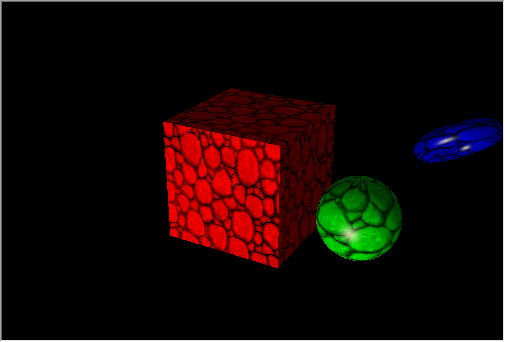### 基于数据的 3D 表面

CDXSurface 类能够依据存储在二维数组中的自定义数据做到表面可视化。 我们查看以下数学函数的示例

`z=sin(2.0*pi*sqrt(x*x+y*y))`

```   virtual bool      Create(const int width,const int height)
{
...
//--- 准备一个数组来存储数据
m_data_width=m_data_height=100;
ArrayResize(m_data,m_data_width*m_data_height);
for(int i=0;i<m_data_width*m_data_height;i++)
m_data[i]=0.0;
//--- 创建一个表面物体
if(!m_surface.Create(m_canvas.DXDispatcher(),m_canvas.InputScene(),m_data,m_data_width,m_data_height,2.0f,
DXVector3(-2.0,-0.5,-2.0),DXVector3(2.0,0.5,2.0),DXVector2(0.25,0.25),
CDXSurface::SF_TWO_SIDED|CDXSurface::SF_USE_NORMALS,CDXSurface::CS_COLD_TO_HOT))
{
m_canvas.Destroy();
return(false);
}
//--- 创建纹理和反射
m_surface.SpecularColorSet(DXColor(1.0,1.0,1.0,1.0));
m_surface.TextureSet(m_canvas.DXDispatcher(),"checker.bmp");
//--- 将表面添加到场景
//--- 成功
return(true);
}

```

• SF_TWO_SIDED 表示如果相机在表面的下方移动，则绘制该表面的上、下方。
• SF_USE_NORMALS 表示计算由定向光源引起的反射时利用法线计算。
• CS_COLD_TO_HOT 设置表面的热图颜色从蓝色至红色，中间由绿色过渡到黄色。

```   void              OnTimer(void)
{
static ulong last_time=0;
static float time=0;
//--- 获取当前时间
ulong current_time=GetMicrosecondCount();
//--- 计算增量
float deltatime=(current_time-last_time)/1000000.0f;
if(deltatime>0.1f)
deltatime=0.1f;
//--- 流逝时间值递增
time+=deltatime;
//--- 记住时间
last_time=current_time;
//--- 参考时间变化计算表面值
for(int i=0; i<m_data_width; i++)
{
double x=2.0*i/m_data_width-1;
int offset=m_data_height*i;
for(int j=0; j<m_data_height; j++)
{
double y=2.0*j/m_data_height-1;
m_data[offset+j]=MathSin(2.0*M_PI*sqrt(x*x+y*y)-2*time);
}
}
//--- 更新数据，绘制表面
if(m_surface.Update(m_data,m_data_width,m_data_height,2.0f,
DXVector3(-2.0,-0.5,-2.0),DXVector3(2.0,0.5,2.0),DXVector2(0.25,0.25),
CDXSurface::SF_TWO_SIDED|CDXSurface::SF_USE_NORMALS,CDXSurface::CS_COLD_TO_HOT))
{
//--- 重新计算 3D 场景，并将其绘制在画布中
Redraw();
}
}

```

MQL5 令您无需使用第三方程序包即可解决重要的算法交易任务：

• 优化包含许多输入参数的复杂交易策略
• 获得优化结果
• 以最方便的三维存储将数据可视化

Step4_Box_Color.mq5 (15.25 KB)
Step7_Animation.mq5 (18.83 KB)
Step9_Texture.mq5 (23.16 KB)
Three_Objects.mq5 (27.9 KB)
3D_Surface.mq5 (24.48 KB)
MQL5.zip (199.48 KB)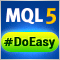轻松快捷开发 MetaTrader 程序的函数库（第 三十三部分）：延后交易请求 - 在特定条件下平仓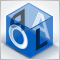在交易中应用 OLAP（第 3 部分）：为开发交易策略而分析报价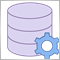应用网络函数，或无需 DLL 的 MySQL：第 I 部分 - 连通器

MetaTrader 5 最近已获增网络函数。 这为程序员开发市场所需产品提供了巨大的机遇。 如今，他们能够实现以前需要动态库支持的功能。 在本文中，我们将以 MySQL 为例研究所有的实现。轻松快捷开发 MetaTrader 程序的函数库（第 三十四部分）：延后交易请求 - 在特定条件下删除和修改订单与持仓